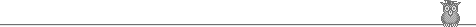Goto: Prof. King's page at Univ. of Florida.    Or: JK Homepage.
Modified: Friday, 12Nov2021     Printed: Tuesday, 07Dec2021
Page: http://squash.1gainesville.com/Include/thispage.shtml
JK focus Articles Fonts
testing Misc Navigation Schedule Teaching StanZas

Michael Dyck's Contradance Index LORs Pamphlets

PAST-COURSES Footnote, good books
SeLo 2021t Plex 2021g
SeLo 2021g DfyQ 2021g
SeLo 2020t, both sections Combinatorics 2017-2018
SeLo 2020g DfyQ 2020g
Algebra.1 2019t SeLo 2019t
NT&Crypto 2019g DfyQ 2019g

#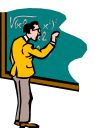Past courses taught…

…by Prof. JLF King
Course pages have exams, final-projects and mathematical handouts. The Teaching Page has a large collection of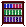Usually Useful Pamphlets. Also available is a chronological list of course descriptions 2003–Now and a list of course-Titles, 1990–Now.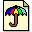Ergodic Theory & Dynamical Systems , [Autumn 2009] . Full-year introductory course in Dynamical Systems with emphasis on Ergodic theory (studies measure-preserving maps of a space to itself) and elementary Topological Dynamics (studies continuous maps of a compact metric-space to itself). As time permits, Symbolic or Differential dynamics may be studied, particularly in the 2nd semester. (See also ET&DS from Aut. 2000.)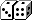Probability & Potential Theory 1, [Autumn 2003]. MAP6472 MWF2 in LIT217.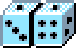Probability & Potential Theory 2, [Spring 2004]. MAP6473 MWF 7 in FLO100. (Griffin-Floyd Hall)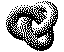Topology One , Autumn 1997. Has elementary results on connectedness, compactness and metric spaces; several were written by my students.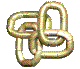Topology Two , the Spring 1998 continuation.

NT1,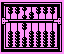Introduction to Number Theory [Spring 2007]. Modular arithmetic, Chinese Remainder Thm, Legendre/Jacobi symbols, Quadratic reciprocity, Fermat SoTS & Lagrange 4-square theorems, basic cryptography (Diffie-Hellman, RSA). Assumes no previous knowledge of Number Theory.

In Spring&Aut. 2006, this ran as a full-year course (using Special topics MAT4930 for the 2nd course-number). The 2nd semester emphasized Cryptography. Again in Spring 2000 & Spring 2001, was a two-semester course.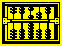Number Theory 2 , [Spring 2001]. This course is a continuation of elementary algebraic number theory. It assumes that the student is comfortable with the notion n mod k, what a prime number is, the Chinese Remainder Theorem, and the Legendre symbol. Roughly it assumes the first half of Strayer's Elementary Number Theory, or parts of the first 3 chapters of An Introduction to the Theory of Numbers by Niven, Zuckerman and Montgomery.NT & Mathematical Cryptography [NT&Crypto] Spring 2016. Assumes only a basic knowledge of modular arithmetic, and the Euler phi-function. Reading the first chapter or two of any standard text, e.g Stayer's text, or Silverman's “Friendly” text.

Full-year course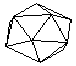Combinatorics I & II, Autumn 2017, Spring 2018.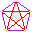Combinatorics I , first semester, Autumn 2012.Combinatorics II , second semester, in Spring 2013.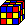Abstract Algebra 1 [Spring 2008 and Aut. 2005] . Group theory, with some discussion of Fields and Rings.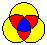Sets and Logic (SeLo) [Aut. 2013]. Teaches students to read and produce proofs, and learn the basic language of modern Mathematics.
See also SeLo 2012 (SeLo) [Spring. 2012]. and SeLo 2011 (SeLo) [Aut. 2011]. which also has Autumn 2009 and Spring 2008.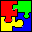Numbers & Polynomials (NaPo) [Spring 2006]. Text: Numbers & Polynomials by Prof. Kermit Sigmon. This course is run Moore Method, meaning that students prove all theorems, with enlightened guidance from the Professor.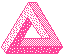Computational Linear Algebra [Aut. 2007]. Introduces Matrices, determinants, the Gauss-Jordan algorithm... .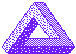(theoretical) Linear Algebra [Aut. 2005]. MAS4105, which is a proof-based course. See also LinA [Aut.2015] and LinA [Aut.2010] and LinA [Aut.2010].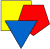Euclidean Geometry. [Spring 2012] A proof-based course covering a superset of: Theorems on Triangles (centroid, in-center, circum-center, ortho-center, Euler-line, Simson-Line), circles (Central-angle thm, Power-of-a-point), ruler/straightedge contructions and dissections of polygons. Matrix multiplication will be introduced for easy descriptions of transformations preserving Euclidean theorems. Time permitting, elem. Projective Geometry will be introduced, since many PG thms are also EG thms.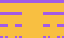ACES (Advanced Calc for Engineers and Scientists). Real Analysis in Euclidean spaces [full-year course.].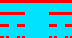ACT (Advanced Calculus, Theoretical). Real Analysis in metric spaces [full-year course.]. This is the AdvCalc for those intending graduate work in Mathematics.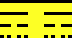Modern Analysis I . A full-year course in Real Analysis. It uses the highly-regarded Baby Rudin text, and covers some Measure Theory.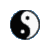Complex analysis , Spring 1999.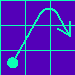Honors Calculus I [Aut. 2001]. Introductory calculus course requiring careful exposition by the students.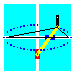Calculus II [Spring 2010]. Careful treatment of 1-dimensional calculus, with emphasis on Taylor's theorem and Taylor series. (Also has notes from Autumn 1995, Calc2.)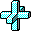Calculus III. Rigorous treatment of multi-dimensional calculus. (Has notes from Autumn 2003&2002, and from Spring 2002&1999.)Elementary Differential Equations [Autumn 2013]. Beginning DiffyQ for the bright, motivated, hard-working student. Also has material from 3 other incarnations of the course.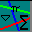Survey of Calculus 2 [Aut. 2000]. Has exams from Spring 2000 and Aut. 1999.